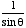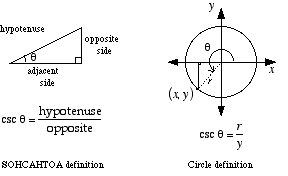index: click on a letter A B C D E F G H I J K L M N O P Q R S T U V W X Y Z A to Z index index: subject areas numbers & symbols sets, logic, proofs geometry algebra trigonometry advanced algebra & pre-calculus calculus advanced topics probability & statistics real world applications multimedia entrieswww.mathwords.com about mathwords website feedback

 cosecant csc cosec The trig function cosecant, written csc θ. csc θ equals. For acute angles, csc θ can be found by the SOHCAHTOA definition, shown below on the left. The circle definition, a generalization of SOHCAHTOA, is shown below on the right. f(x) = csc x is a periodic function with period 2π.See also